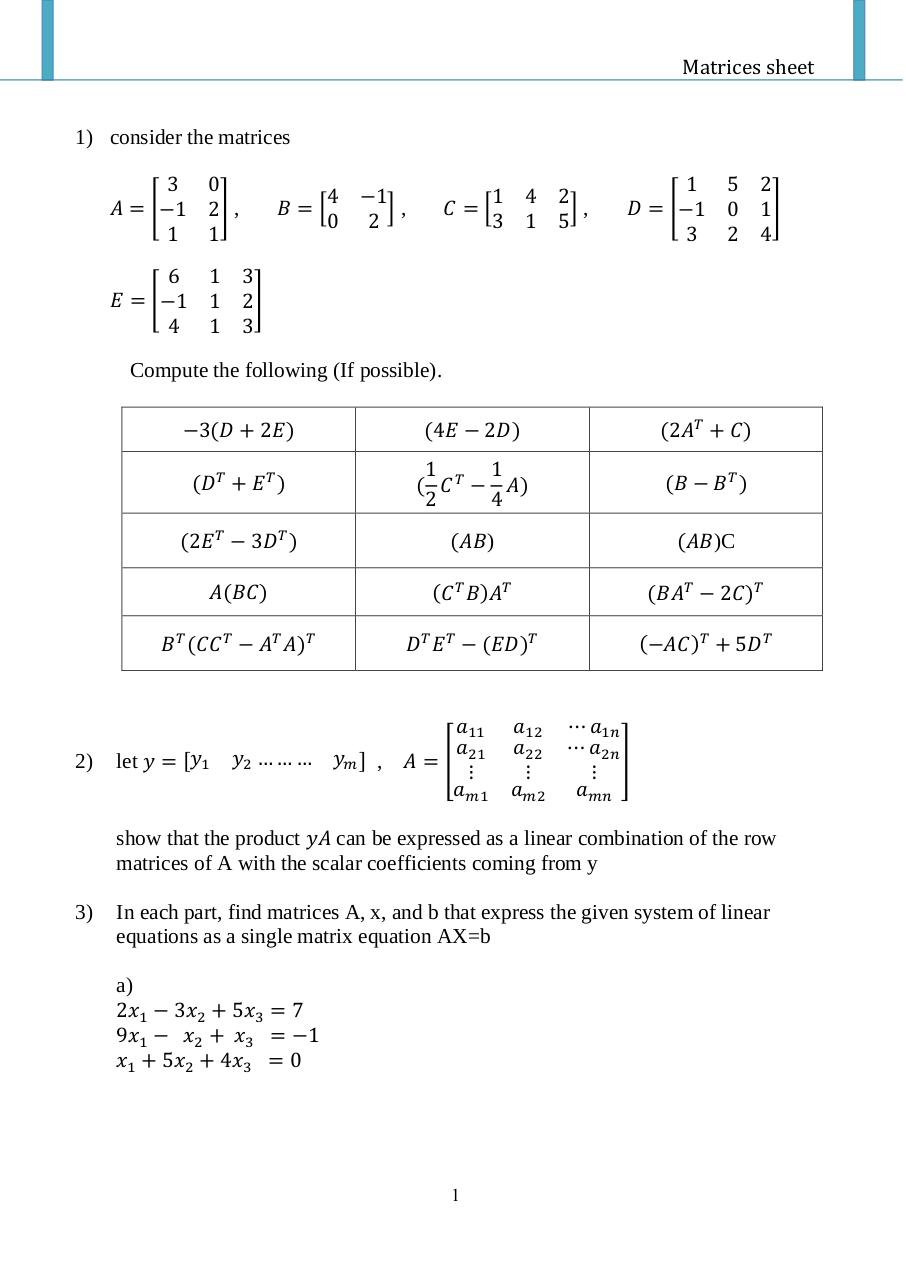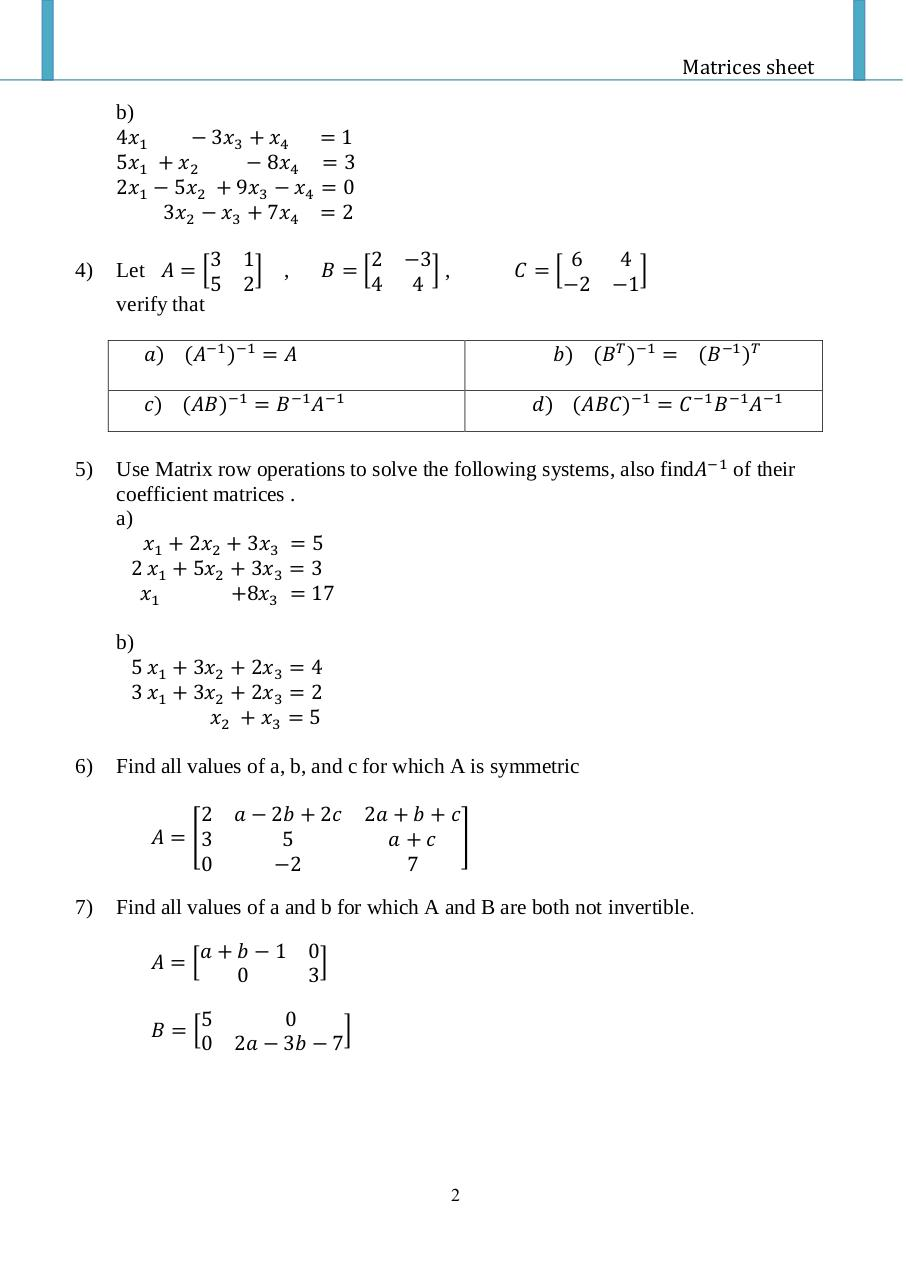# PDF Archive

Easily share your PDF documents with your contacts, on the Web and Social Networks.

## sheet 2 Autosaved .pdf

Original filename: sheet-2-Autosaved.pdf
Title: Matrices sheet
Author: mufic

This PDF 1.5 document has been generated by Microsoft® Word 2013, and has been sent on pdf-archive.com on 06/11/2015 at 09:11, from IP address 41.37.x.x. The current document download page has been viewed 292 times.
File size: 308 KB (2 pages).
Privacy: public file### Document preview

Matrices sheet
1) consider the matrices
3 0
𝐴 = [−1 2] ,
1 1
6 1
𝐸 = [−1 1
4 1

4 −1
𝐵=[
],
0 2

1
𝐶=[
3

4 2
],
1 5

1
𝐷 = [−1
3

5 2
0 1]
2 4

3
2]
3

Compute the following (If possible).

2)

−3(𝐷 + 2𝐸)

(4𝐸 − 2𝐷)

(2𝐴𝑇 + 𝐶)

(𝐷𝑇 + 𝐸 𝑇 )

1
1
( 𝐶 𝑇 − 𝐴)
2
4

(𝐵 − 𝐵𝑇 )

(2𝐸 𝑇 − 3𝐷𝑇 )

(𝐴𝐵)

(𝐴𝐵)C

𝐴(𝐵𝐶)

(𝐶 𝑇 𝐵)𝐴𝑇

(𝐵𝐴𝑇 − 2𝐶)𝑇

𝐵𝑇 (𝐶𝐶 𝑇 − 𝐴𝑇 𝐴)𝑇

𝐷𝑇 𝐸 𝑇 − (𝐸𝐷)𝑇

(−𝐴𝐶)𝑇 + 5𝐷𝑇

let 𝑦 = [𝑦1

𝑎11
𝑦2 … … … 𝑦𝑚 ] , 𝐴 = [ 𝑎21

𝑎𝑚1

𝑎12
𝑎22

𝑎𝑚2

⋯ 𝑎1𝑛
⋯ 𝑎2𝑛
]

𝑎𝑚𝑛

show that the product 𝑦𝐴 can be expressed as a linear combination of the row
matrices of A with the scalar coefficients coming from y
3)

In each part, find matrices A, x, and b that express the given system of linear
equations as a single matrix equation AX=b
a)
2𝑥1 − 3𝑥2 + 5𝑥3 = 7
9𝑥1 − 𝑥2 + 𝑥3 = −1
𝑥1 + 5𝑥2 + 4𝑥3 = 0

1

Matrices sheet
b)
4𝑥1
− 3𝑥3 + 𝑥4 = 1
5𝑥1 + 𝑥2
− 8𝑥4 = 3
2𝑥1 − 5𝑥2 + 9𝑥3 − 𝑥4 = 0
3𝑥2 − 𝑥3 + 7𝑥4 = 2
4)

Let 𝐴 = [

3 1
] ,
5 2

𝐵=[

2 −3
],
4 4

𝐶=[

6
4
]
−2 −1

verify that
𝑎) (𝐴−1 )−1 = 𝐴

𝑏) (𝐵𝑇 )−1 = (𝐵−1 )𝑇

𝑐) (𝐴𝐵)−1 = 𝐵−1 𝐴−1
5)

𝑑) (𝐴𝐵𝐶)−1 = 𝐶 −1 𝐵−1 𝐴−1

Use Matrix row operations to solve the following systems, also find𝐴−1 of their
coefficient matrices .
a)
𝑥1 + 2𝑥2 + 3𝑥3 = 5
2 𝑥1 + 5𝑥2 + 3𝑥3 = 3
𝑥1
+8𝑥3 = 17
b)
5 𝑥1 + 3𝑥2 + 2𝑥3 = 4
3 𝑥1 + 3𝑥2 + 2𝑥3 = 2
𝑥2 + 𝑥3 = 5

6)

Find all values of a, b, and c for which A is symmetric
2 𝑎 − 2𝑏 + 2𝑐
𝐴 = [3
5
0
−2

7)

2𝑎 + 𝑏 + 𝑐
𝑎+𝑐 ]
7

Find all values of a and b for which A and B are both not invertible.
𝐴=[

𝑎+𝑏−1 0
]
0
3

5
𝐵=[
0

0
]
2𝑎 − 3𝑏 − 7

2